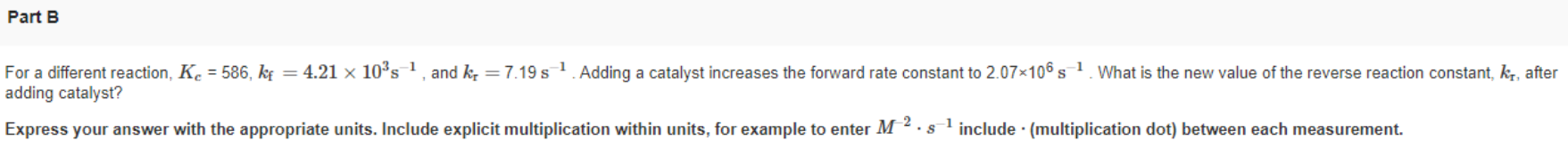# For a different reaction, Kc = 586, kf = 4.21x10^3 s^-1, and kr = 7.19 s^-1. Adding a catalyst increases the forward rate constant to 2.07x10^6s^-1. What is the new value of the reverse reaction constant, kr, after adding catalyst? Express your answer with the appropriate units. Include explicit multiplication within units, for example to enter M^-2 . s^-1 include . (multiplication dot) between each measurement.